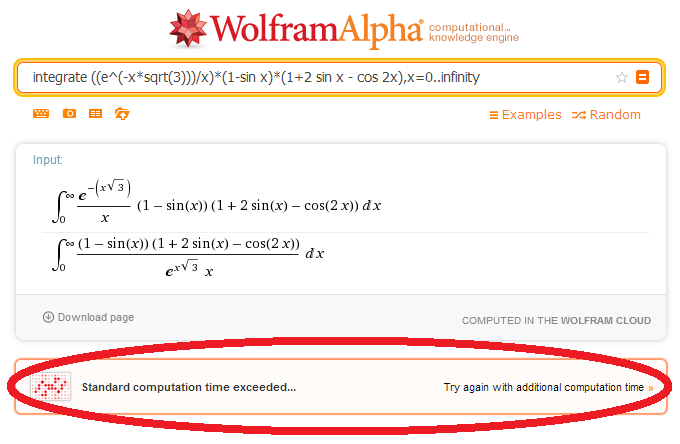# Wolfram Alpha Can't Do This, Can You?

Calculus Level 5Today, when I was in my college library, I saw two engineer students were desperate to do their calculus homework. They became more frustrated when realized even Wolfram Alpha failed to answer it. I took a look their homework in case I could solve it. This is their homework:

$\int_0^\infty\frac{e^{-x\sqrt{3}}}{x}\left(1-\sin x\right)\left(1+2\sin x-\cos 2x\right)\;dx=\;...$

Could you help them out?

×

Problem Loading...

Note Loading...

Set Loading...